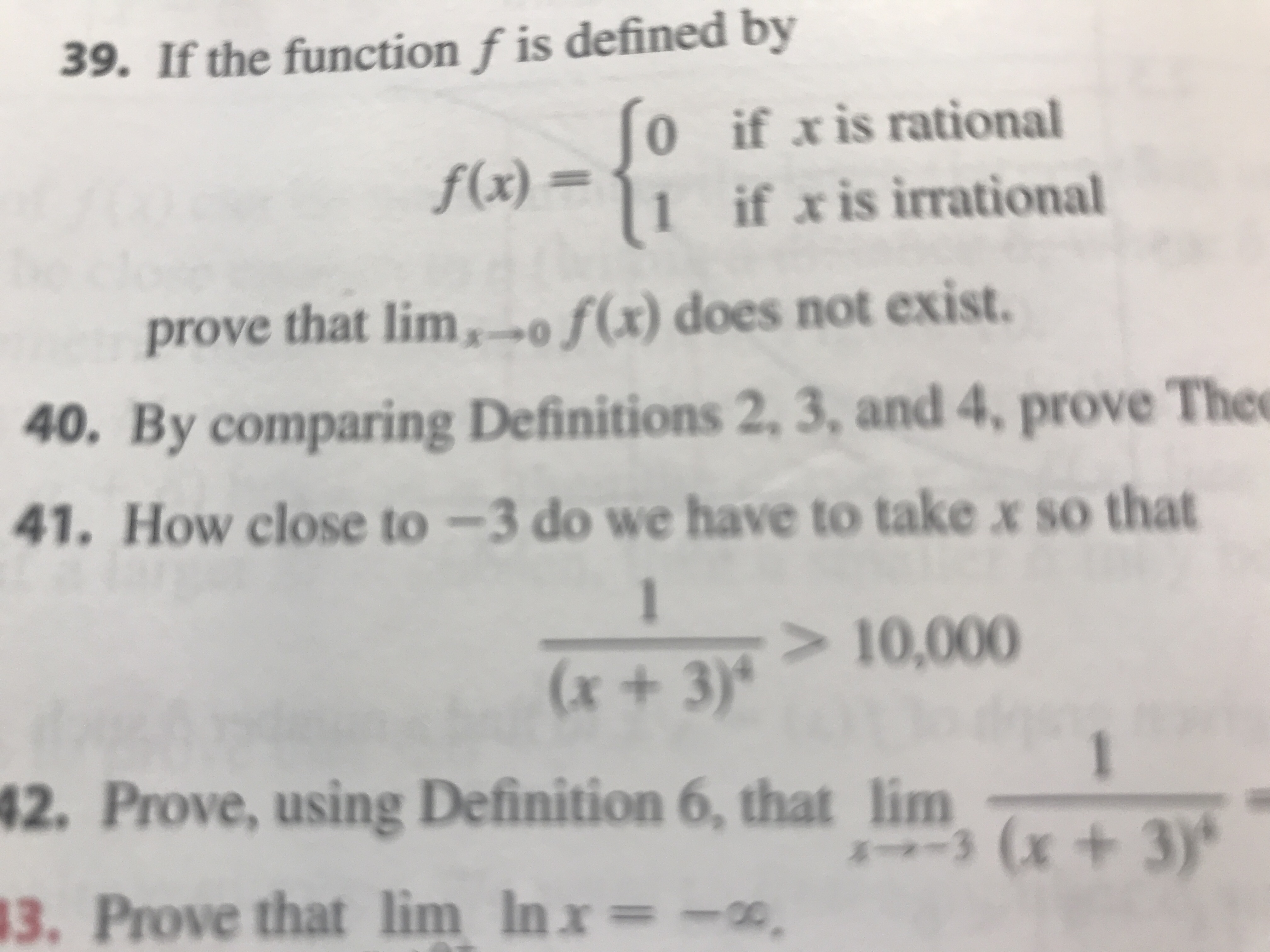# 39. If the function f is defined by 0 if xis rational f(x) = if x is irrational prove that lim,o f(x) does not exist. 40. By comparing Definitions 2, 3, and 4, prove Thee 41. How close to -3 do we have to take x so that 1 > 10,000 (x+3)' 1 42. Prove, using Definition 6, that lim(x+3) 3. Prove that lim Inx = -co

Question

Number 41help_outlineImage Transcriptionclose39. If the function f is defined by 0 if xis rational f(x) = if x is irrational prove that lim,o f(x) does not exist. 40. By comparing Definitions 2, 3, and 4, prove Thee 41. How close to -3 do we have to take x so that 1 > 10,000 (x+3)' 1 42. Prove, using Definition 6, that lim(x+3) 3. Prove that lim Inx = -co fullscreen